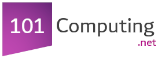Start

End
Create a program that asks the user to enter a whole number.
The program will then display the times table for this number.
number = int(input("Type a number:"))
print(str(number) + " Times Table:")
for i in range(1,11):
product = number * i
print(str(number) + " x " + str(i) + " = " + str(product))